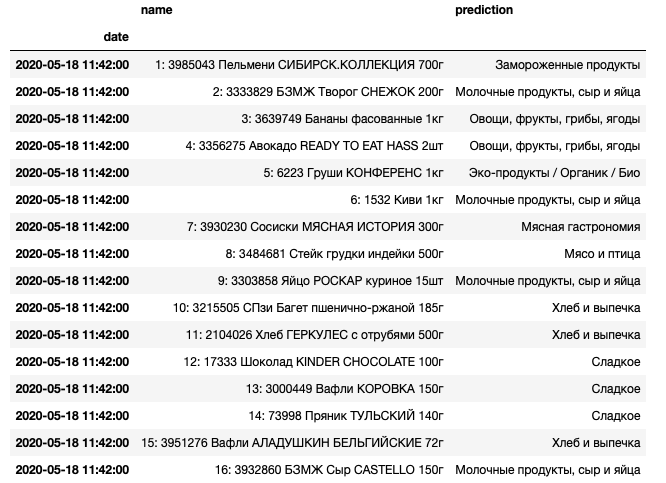# Predicting category of products by name from Russian Food Stores

Время чтения текста – 9 минут

This article is a continuation of our series about analyzing data on consumer products: «Collecting data from hypermarket receipts on Python» and «Parsing the data of site’s catalog, using Beautiful Soup and Selenium». We are going to build a model that would classify products by name in a till receipt. Till receipts contain data for each product bought, but it doesn’t provide us a summary of how much were spent on Sweets or Dairy Foods in total.

## Data Wrangling

Load data from our .csv file to a Pandas DataFrame and see how it looks:

Did you know that we can emulate human behavior to parse data from a web-catalog? More details about it are in this article: «Parsing the data of site’s catalog, using Beautiful Soup and Selenium»

``````import pandas as pdAs you can see, the DataFrame contains even more than we need for predicting the category of products by name. So we can drop() columns with prices and weights, and rename() the remaining ones:

``````sku.drop(columns=['Unnamed: 0', 'Weight','Price'],inplace=True)
sku.rename(columns={"SKU": "SKU", "Category": "Group"},inplace=True)Group the products by its category and count them up with the following methods:

``sku.groupby('Group').agg(['count'])``We will train our predictive model on this data so that it could identify the product category by name. Since the DataFrame includes product names mainly in Russian, the model won’t make predictions properly. The Russian language contains a lot of prepositions, conjunctions, and specific speech patterns. We want our model to distinguish that «Мангал с ребрами жесткости» («Brazier with strengthening ribs» ) and «Мангал с 6 шампурами» («Brazier with 6 skewers») belongs to the same category. With this is my we need to clean up all the product names, removing conjunctions, preposition, interjections, particles and keep only word bases with the help of stemming.

A stemmer is a tool that operates on the principle of recognizing “stem” words embedded in other words.

``````import nltk
from nltk.corpus import stopwords
from pymystem3 import Mystem
from string import punctuation

In our case will be using the pymystem3 library developed by Yandex. Product names in our DataFrame may vary from those ones you could find in supermarkets today. So first, let’s improve the list of stop words that our predictive model will ignore.

``````mystem = Mystem()
russian_stopwords = stopwords.words("russian")
russian_stopwords.extend(['лента','ассорт','разм','арт','что', 'это', 'так', 'вот', 'быть', 'как', 'в', '—', 'к', 'на'])``````

Write a function that would preprocess our data and extract the word base, remove punctuation, numerical signs, and stop words. The following code snippet belongs to one Kaggle kernel.

``````def preprocess_text(text):
text = str(text)
tokens = mystem.lemmatize(text.lower())
tokens = [token for token in tokens if token not in russian_stopwords\
and token != " " \
and len(token)>=3 \
and token.strip() not in punctuation \
and token.isdigit()==False]
text = " ".join(tokens)
return text``````

See how it works:

An extract from Borodino (Russian: Бородино), a poem by Russian poet Mikhail Lermontov which describes the Battle of Borodino.

``preprocess_text("Мой дядя самых честных правил, Когда не в шутку занемог, Он уважать себя заставил И лучше выдумать не мог.")``

Transformed into:

``'дядя самый честный правило шутка занемогать уважать заставлять выдумывать мочь'``

Everything works as expected – the result includes only word stems in lower case with no punctuation, prepositions or conjunctions. Let’s apply this function to a product name from our DataFrame:

``````print(‘Before:’, sku['SKU'])
print(‘After:’, preprocess_text(sku['SKU']))``````

Preprocessed text:

``````Before: Фисташки соленые жареные ТМ 365 дней
After: фисташка соленый жареный день``````

The function works fine and now we can apply it to the whole column, and create a new one with processed names:

``````sku['processed']=sku['SKU'].apply(preprocess_text)## Building our Predictive Model

We will be using CountVectorizer to predict the product category, and Naive Bayes Classifier.
CountVectorizer will tokenize our text and build a vocabulary of known words, while Naive Bayes Classifier allows us to train our model on a DataFrame with multiple classes. We will also need TfidfTransformer for computing words count (term frequency). As we want to chain these steps, let’s import the Pipeline library:

``````import pandas as pd
from sklearn.model_selection import train_test_split
from sklearn.feature_extraction.text import CountVectorizer
from sklearn.feature_extraction.text import TfidfTransformer
from sklearn.naive_bayes import MultinomialNB
from imblearn.pipeline import Pipeline``````

Separate our targets, Y (categories) from the predictors, X (processed product names). And split the DataFrame into Test and Training sets, allocating 33% of samples for testing.

``````x = sku.processed
y = sku.Group
X_train, X_test, y_train, y_test = train_test_split(x, y, test_size=0.33)``````

Add the following methods to our pipeline:

• CountVectorizer() – returns a matrix of token counts
• TfidfTransformer() – transforms a matrix into a normalized tf-idf representation
• MultinomialNB() – an algorithm for predicting product category
``````text_clf = Pipeline([('vect', CountVectorizer(ngram_range=(1,2))),
('tfidf', TfidfTransformer()),
('clf', MultinomialNB())])``````

Fit our model to the Training Dataset and make predictions for the Test Dataset:

``````text_clf = text_clf.fit(X_train, y_train)
y_pred = text_clf.predict(X_test)``````

Evaluate our predictive model:

``print('Score:', text_clf.score(X_test, y_test))``

The model predicts correctly 90% of the time.

``Score: 0.923949864498645``

## Validate our model with the real-world data

Let’s test how good our model performs on real-world data. We’ll refer to the DataFrame from our previous article, «Collecting data from hypermarket receipts on Python», and preprocess the product names:

``````my_products['processed']=my_products['name'].apply(preprocess_text)Pass the processed text to the model and create a new column that would hold our predictions:

``````prediction = text_clf.predict(my_products['processed'])
my_products['prediction']=prediction
my_products[['name', 'prediction']]``````

Now, the DataFrame looks the following:Calculate the spendings for each product category:

``my_products.groupby('prediction').sum()``Overall, the model seems to be robust in predicting that sausages fall under meat products, quark is a dairy product, baguette belongs to bread and pastries. But sometimes it misclassifies kiwi as a dairy product and pear as an eco-product. This is probably because these categories include many products are «with the taste of pear» or «with the taste of kiwi», and the algorithm makes predictions based on the prevailing group of products. This is a well-known issue of unbalanced classes, but it can be addressed by resampling the DataSet or choosing proper weights for our model.

Share
Send
Pin
Popular